# Multiplying 2 and 3-Digit by 1-Digit Numbers

Times up!
Times up! You may finish answering the current question and then proceed to your score!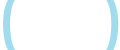Sorry, you have used all of your available hints for this lesson.
Practice Limit Reached

You've reached your daily practice limit of 25 questions.

Are you over 18 years old?
Award InformationAwarded toQuestion HintChoose Family Member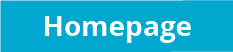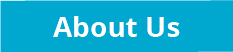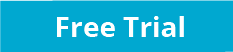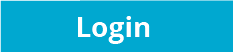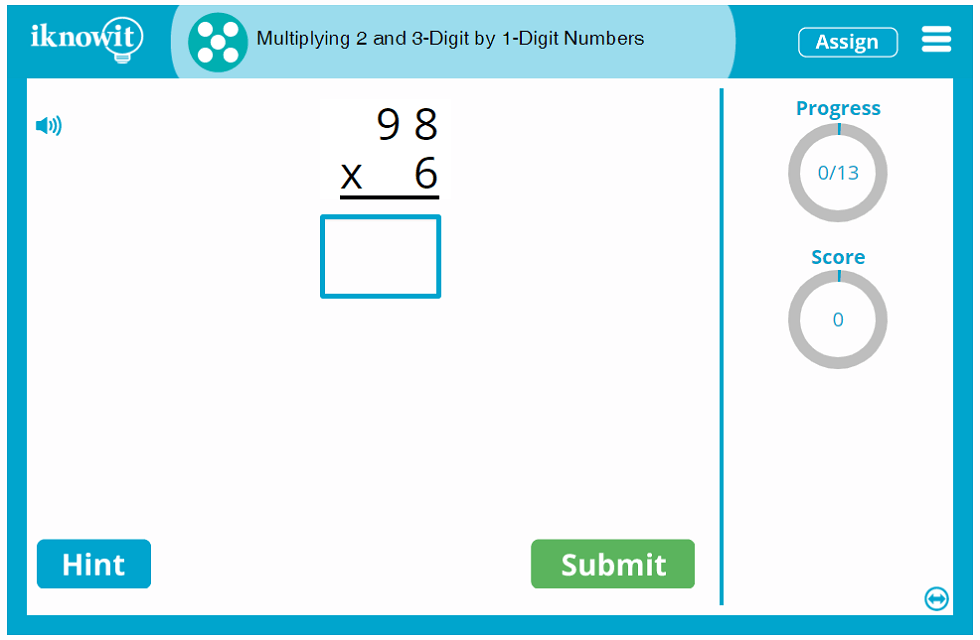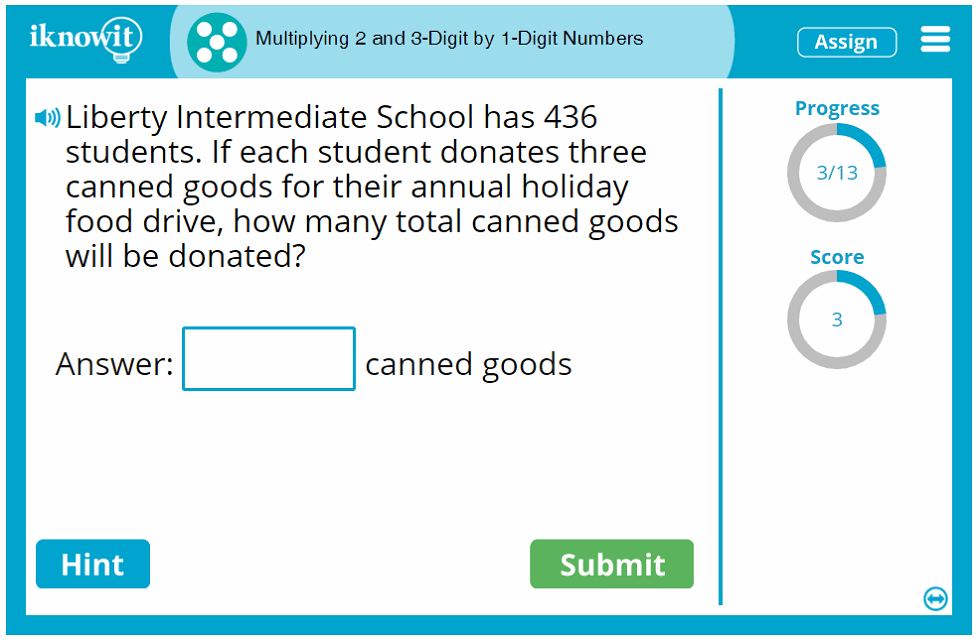## Interactive Math Practice - Multiplying 2 and 3 Digit Numbers by 1 Digit Numbers

It's time to practice basic multiplication skills! In this fifth grade-level multiplication lesson, students will practice multiplying 2- and 3-digit numbers by 1-digit numbers. The multiplication problems are presented in several formats: vertical multiplication problems, horizontal multiplication problems, and multiplication word problems. Here are some examples of math problems students may be asked in this online math practice activity: "22 x 7 =", "876 x 3 =", and "Discovery Charter School's PTA was hosting an ice cream social. They ordered four boxes of 480 plastic spoons for the event. How many spoons did the PTA order in all?"

If students need a little extra help answering their multiplication problems, they can click on the "Hint" button on their practice screen. They will be prompted to take the first step in solving the problem, multiplying the ones. If students answer a question incorrectly, they will be showed a detailed explanation page with the correct answer, including a custom graphic that provides step-by-step instructions for how to solve the multiplication problem correctly, so students can learn from their mistakes and improve their skills.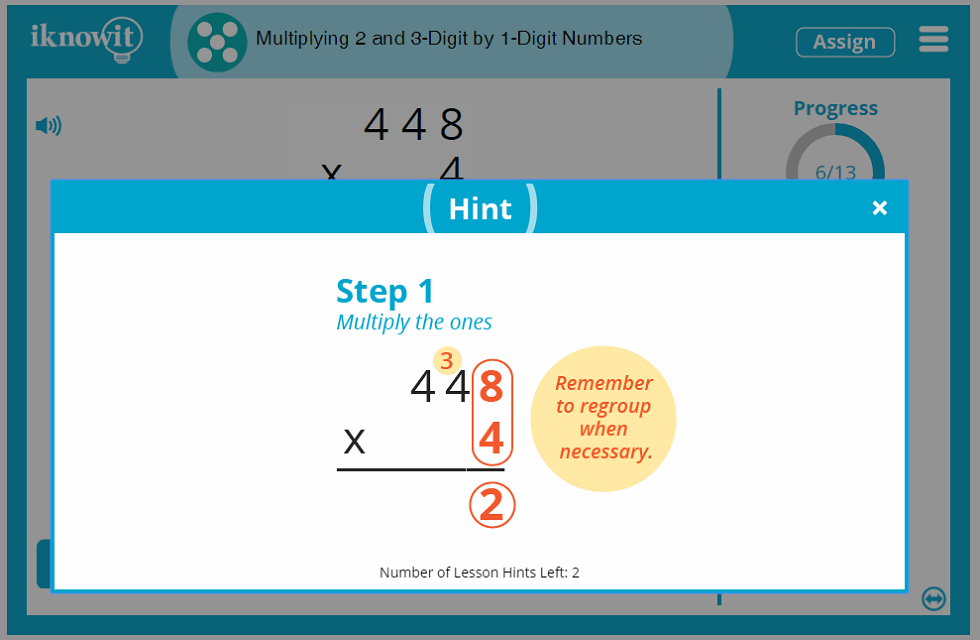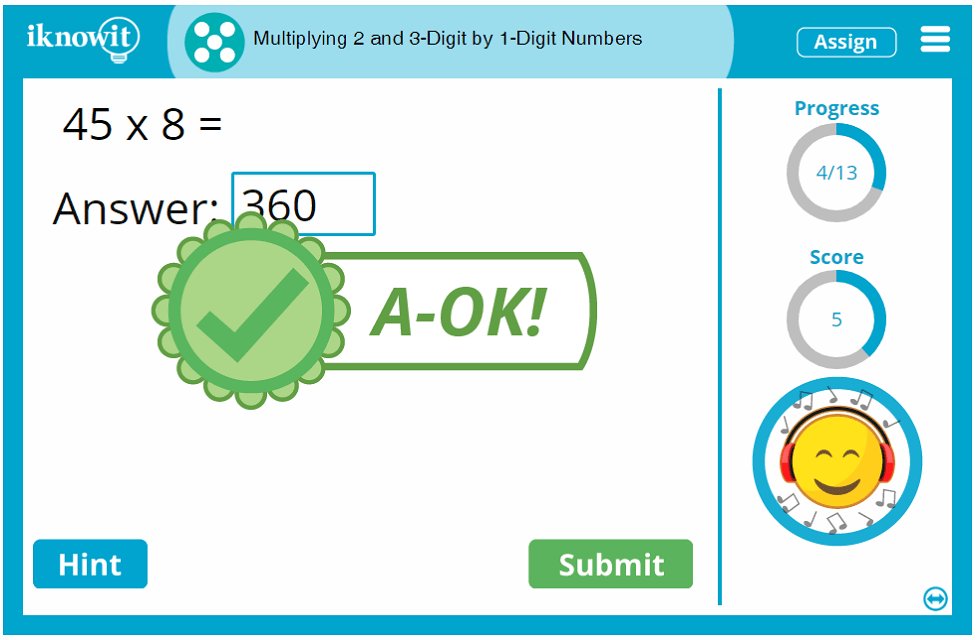## How does iKnowIt.com work?

As with all the interactive math practice activities on "I Know It," this basic multiplication lesson includes several features that help students make the most out of their math practice time. A progress-tracker in the upper-right corner of the screen shows students how many math problems they've solved in the lesson so far, and how many are left to go. A score-tracker beneath that shows them how many math problems they have solved correctly so far. A speaker icon in the upper-left corner of the screen gives students the option of having the question read aloud to them in a clear voice. This option is great for ESL/ELL students or students who prefer auditory learning.

We hope when you try out this fifth grade multiplication lesson with your class, you and your students will enjoy the bright and colorful design of our interactive math practice program, including its kid-friendly features, whimsical animated characters, and motivating reward system. Be sure to explore the hundreds of other math topics available on "I Know It."

## Free Trial and Membership Options

Sign up for a free sixty-day trial of iKnowIt.com so your class can try out this basic multiplication lesson at no cost! In the free trial mode, your students will be able to play any math game on our website for free, but they will be limited to a total of twenty-five questions per day across all “I Know It” math lessons. For unlimited access to the website, you will need to become a paying member.

Here are some of the benefits you will enjoy as a member of iKnowIt.com: You can create a class roster and add your students to it; you can assign individual user names and passwords to each student; you can give different students different lessons to complete based on their individual needs and skills; you can change lesson settings at any time; and you can track your students' progress through their lessons, including downloading, emailing, and printing student progress reports.

We think your students will find it easy and fun to use the student interface of iKnowIt.com! When they log into the site with their unique user name and password, they will be shown a kid-friendly homepage, where they will find their lessons to complete under the "My Assignments" section. They will also have the option to explore other lessons at their grade level, and if you choose to let them, they can explore lessons at other grade levels too. (Grade levels appear as "Level A" through "Level E" in the student mode.)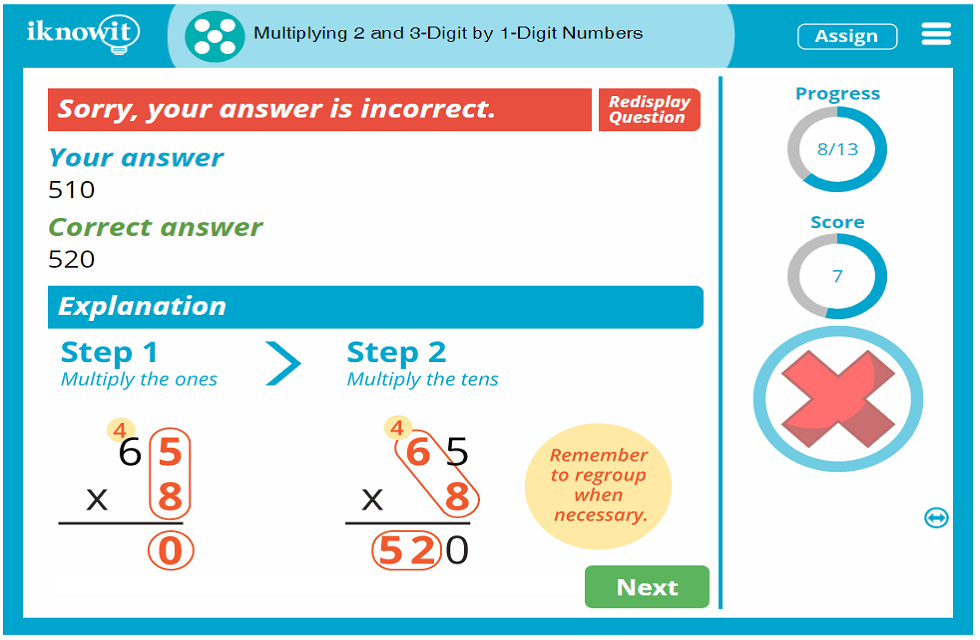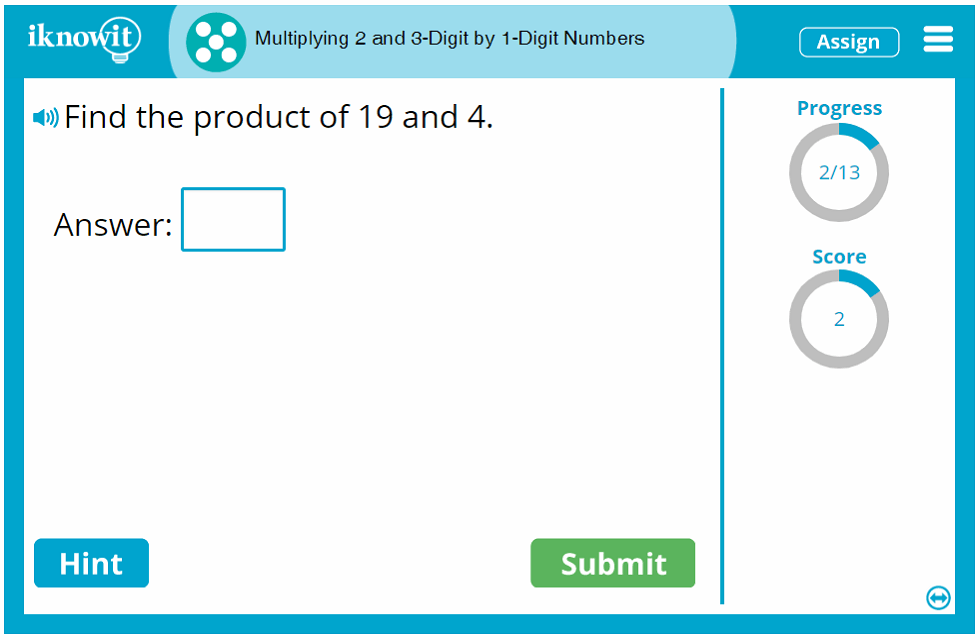## Level

This Level E multiplication lesson may be ideal for fifth grade students.

## Common Core Standard

5.NBT.B.5
Number And Operations In Base Ten

Students will be able to perform operations with multi-digit whole numbers and with decimals to hundredths.

## You might also be interested in...

Multiplying 4-Digit by 1-Digit Numbers (Level E)
In this fifth grade-level math lesson, students will practice multiplying 4-digit by 1-digit numbers. Students will solve horizontal multiplication problems, vertical multiplication problems, and multiplication word problems.

Multiplying 2-Digit by 2-Digit Numbers (Level E)
Students will practice multiplication skills in this fifth grade math lesson. Math problems include vertical multiplication problems, horizontal multiplication problems, and multiplication word problems.